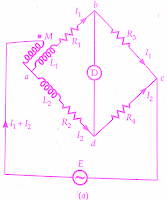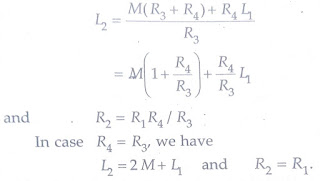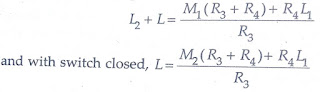# Measurement of Mutual Inductance By Heaviside Bridge

0
123

## Heaviside Mutual Inductance Bridge:

#### Heaviside Bridge measures mutual inductance in terms of a known self-inductance.The same bridge, slightly modified, was used by Campbell to measure a self-inductance in terms of a known mutual inductance.### Measurement of Mutual Inductance By Heaviside Bridge:

#### At balance voltage drop between b and c must equal the voltage drop between d and c.Also, the voltage drop across a-b-c must equal the voltage drop across a-d-c.Thus we have the following equations at balance.#### Heaviside Bridge method can be used for measurement of self-inductance.Supposing L2 is the self-inductance to be determined. From above equations, we get### Campbell’s Modification of Heaviside Bridge:

#### The below figure shows a modified Heaviside bridge.This modification is due to Campbell.This is used to measure a self-inductance in terms of a mutual inductance.In this case, an additional balancing coil R is included in arm a-d in series with inductor under test.An additional resistance r is put in arm a-b.Balance is obtained by varying M and r.A short circuiting switch is placed across the coil R2, L2 under measurement.Two sets of readings are taken one with switch being open and the other with switch being closed.Let values of M and r, be M1 and r1 with switch open, and M2 and r2 with switch closed.#### With switch open from above equation we have,### Heaviside Campbell Equal Ratio Bridge:

#### At balance      I1R3 = I2R4      but  R3 = R4and therefore      I1 = I2 = I/2     as      I = I1 + I2Writing the other equation for balance#### Thus the magnitude of inductance measured with this method is twice the range of the mutual inductor.The values calculated above include the effects of leads etc.In order to eliminate these effects, we take two readings with the switch open circuited and another with the switch closed.Let M1, r1 be the readings of M,r with open circuit and M2, r2 with the short circuit.R1 = (r1-r2) and  L1 = 2(M1-M2)

READ HERE  Torque Equation of Induction Relay - its Derivation